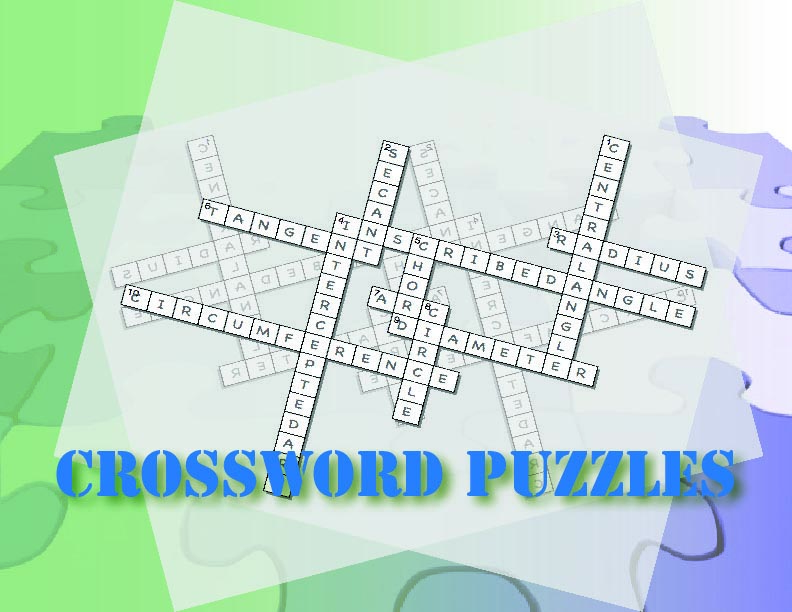# Unit: Introduction to Fractions### Key topics covered in include:

• Properties of fractions
• Fractions in simplest form
• Unit fractions
• Proper and improper fractions
• Equivalent fractions

### The suggested timeframe for each lesson can be adapted to suit your needs.### Days 1-2: Speaking Math

Introduction of key vocabulary around fractions. This is a gamified experience with word puzzles and other games.

Introduce these terms and definitions

• Fraction
• Numerator
• Denominator
• Equivalent Fraction
• Simplest form
• Proper Fraction
• Improper Fraction
• Mixed Number
• Least Common Denominator
• Unit Fraction

Media4Math Resources:

Goal: A low-stress intro to fractions vocabulary that will be used throughout this unit.### Days 3-4: Building Blocks

Students can identify and write fractions. From this students are shown how to write fractions in simplest form.

Media4Math Resources:

Goal: To develop a concrete understanding of fractions in simplest form.### Days 5-6: Putting it Together

Students learn about unit fractions, proper fractions, and improper fractions.

Media4Math Resources:

Goal: To expand on the definition of a fraction to include unit, proper, improper, and equivalent fractions.### Days 7-8: Show What You Know

Use the tutorial and worksheet to develop and practice the skills of identifying and generating equivalent fractions. This student module includes assessment items. You can assign this module to individual students and create a dashboard to track student progress.

Media4Math Resources:

Goal: To build on previous understanding to identify and generate equivalent fractions.